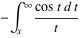## Making simple NAG functions available to Python

April 1st, 2009 | Categories: NAG Library, programming, python | Tags:

This is part 1 of a series of articles devoted to demonstrating how to call the Numerical Algorithms Group (NAG) C library from Python. Click here for the index to this series.

Our first NAG/Python example is going to use the NAG function s13acc which calculates the Cosine integral Ci(x) defined asAccording to the NAG documentation, the algorithm used is based on several Chebyshev expansions. So, let’s see what we need to do to access this function from Python.

Assuming that you have installed the cllux08dgl implementation of the NAG C library, you should find that the following Python code evaluates Ci(x) for x=0.2.
 #!/usr/bin/env python from ctypes import *

libnag = cdll.LoadLibrary("/opt/NAG/cllux08dgl/lib/libnagc_nag.so.8") nag_cos_integral = libnag.s13acc nag_cos_integral.restype=c_double

x = c_double(0.2) ifail=c_int(0)

y= nag_cos_integral( x, ifail ) print "x= %s, cos_integral= %s" % (x.value,y) 

Save (or download) this to a file called s13acc.py, make it executable and run it by typing the following at a bash prompt.
 chmod +x ./s13acc.py ./s13acc.py 
If all has gone well then you should get following result
 x= 0.2, cos_integral= -1.04220559567 
Since this our first piece of NAG/Python code let’s go through it line by line.
 from ctypes import * 
This simply imports everything from the standard ctypes module and makes it available in the global namespace.
 libnag = cdll.LoadLibrary("/opt/NAG/cllux08dgl/lib/libnagc_nag.so.8") nag_cos_integral = libnag.s13acc 
The first line loads the NAG library using the cldll.LoadLibrary() function and associates it with an object I have named libnag whereas the second line pulls the function s13acc out of libnag and associates it with an object having the more user-friendly name of nag_cos_integral.

This is the least portable part of the code. If you are using a different implementation of the NAG C library then you will need to change the path in the LoadLibrary function.
 nag_cos_integral.restype=c_double 
The ctypes module provides us with a set of C compatible data-types such as c_int, c_double, c_char and so on, and we have to use these when we interact with C functions. The object nag_cos_integral represents the NAG C function we wish to call and by default ctypes assumes that the return type of all C functions is of type c_int unless we tell it otherwise.

We know from the documentation for s13acc that its return type is double and so here we are using the restype method function to set the return type of nag_cos_integral to c_double.
 x = c_double(0.2) ifail=c_int(0) 
Here, we are setting up our input arguments. x is straightforward enough but the observant reader will notice that there is something fishy going on with the ifail parameter. The NAG documentation tells us that the ifail parameter of s13acc should be a pointer to a NagError structure but structures are a complication that I don’t want to deal with here so I have cheated and used the fact that the integer 0 is defined as NAGERR_DEFAULT via an enumeration in the C library header file. In short, this means that I don’t have to use a NagError structure if I don’t want to. Full details can be found in section 2.6 of the Essential Introduction to the NAG C Library.

We’ll look at how to use the NagError structure properly via Python in a later article for those who need it.
 y= nag_cos_integral( x, ifail ) 
Here we pass our input parameters to the nag_cos_integral function and store the result in y. When I was working all of this out for the first time, I expected y to be of type c_double. After all, we earlier used restype to set the return type of nag_cos_integral to c_double so that’s what I expected to get but it turns out that y is a plain old python double. This is clearly explained in the ctypes documentation and I quote the relevant section below

‘Fundamental data types, when returned as foreign function call results, or, for example, by retrieving structure field members or array items, are transparently converted to native Python types. In other words, if a foreign function has a restype of c_char_p, you will always receive a Python string, not a c_char_p instance.’

 print "x= %s, cos_integral= %s" % (x.value,y) 
Finally, we actually print the output. Since x is a c_double we need to use the value attribute to ensure that 0.2 gets printed rather than c_double(0.2). The variable y is a python double as explained earlier so it needs no special treatment.

That’s it for this article in the series. Next time I’ll give an example using this function along with matplotlib to produce some more interesting output before moving onto callback functions.

As always, questions and comments are welcomed.